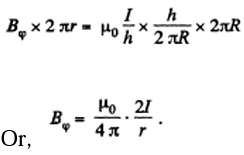# I.E. Irodov Solutions on Constant Magnetic Field. Magnetics

Magnetics is an important chapter in Physics that mainly deals with the magnetic field and magnets. The chapter comprises various numerical based on the syllabus and also the practical applications. I.E. Irodov Physics provides the solutions to different varieties of numerical. Constant Magnetic Field Magnetics is a necessary resource element that is needed by all students to score good marks in JEE Main and other entrance examinations. It is one of the best ways to study and practice for physics examinations to score high. The problems asked here are mainly based on current in a circuit, voltage, movement of charge and so on.

The detailed stepwise explanation for every solution makes it easier for the student to understand. This chapter mainly focuses on formulas and equations based on the magnetic field and its importance. It helps the students to analyse how to apply the theoretical knowledge. The problems given here focus on the variation of magnetic field, with the respective materials and their importance. The concept explained here is crystal clear and goes hand to hand with the syllabus. One can get access to all sorts of problems based on each and every formula, equations and method. The questions asked are at a standard level for a student of Class 12 to solve. Solving these types of problems builds the confidence of students and enables them to have a grip over the subject.

### I.E. Irodov Solutions on Constant Magnetic Field. Magnetics

1. A current I = 1.00 A circulates in a round thin wire loop of radius R = 100 mm. Find the magnetic induction

1. (a) at the centre of the loop;

2. (b) at the point lying on the axis of the loop at a distance centre.

Solution:

1. (a) From Biot Savart’s law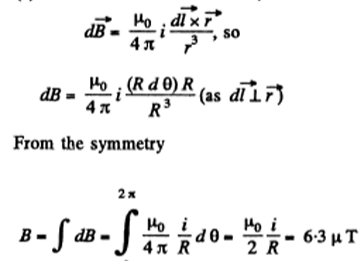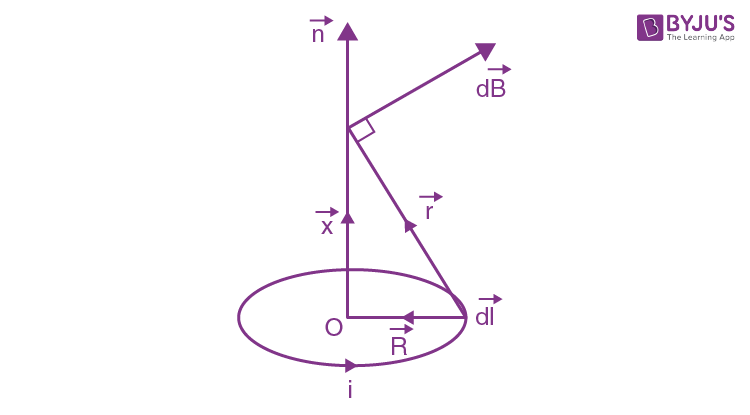(b) From Biot Savart law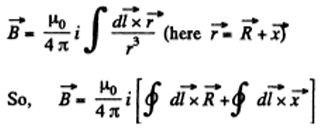Since $\vec{x}$ is a constant and $\left | \vec{R} \right |$ is also constant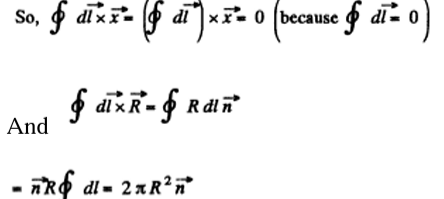Here $\vec{n}$ is a unit vector perpendicular to the plane containing the current loop and in the direction of $\vec{x}$.

Thus we get

$\vec{B}=\frac{\mu _{0}}{4\pi }\frac{2\pi R^{2}i}{(x^{2}+ R^{2})\frac{3}{2}}\vec{n}$

2. A current I flows along a thin wire shaped as a regular polygon with n sides which can be inscribed into a circle of radius R. Find the magnetic induction at the centre of the polygon. Analyse the obtained expression?

Solution:

1. As $\angle AOB=\frac{2\pi }{n}$, OC or perpendicular distanc of any segment from centre equals $R\cos \frac{\pi }{n}$ Now magnetic induction at O, due to die right current carrying element AB.

$\frac{\mu _{0}}{4\pi }\frac{i}{R\cos\frac{\pi }{n}}2\sin\frac{\pi }{n}$

From BiotSavart’s law, the magnetic field at O due to any section such as AB is perpendicular to the plane of the figure and has the magnitude.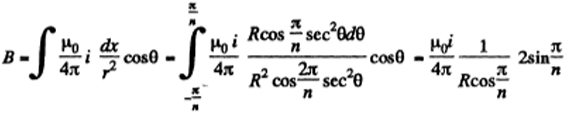As there are n number of sides and magnetic induction vectors, due to each side at O, are equal in magnitude and direction. So,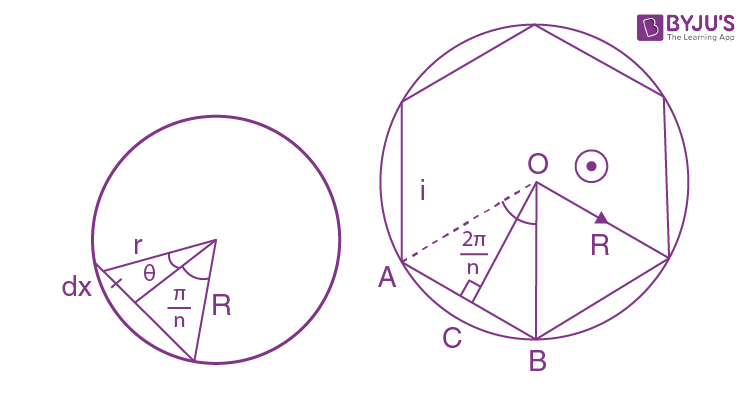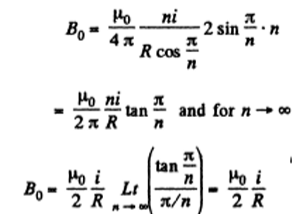3. Find the magnetic induction at the centre of a rectangular wire frame whose diagonal is equal to d = 16 cm and the angle between the diagonals is equal to φ = 30°; the current flowing in the frame equals I = 5.0 A

Solution:

1. We know that magnetic induction due to a straight current-carrying wire at any point, at a perpendicular distance from it is given by

$\vec{B}=\frac{\mu _{0}}{4\pi }\frac{i}{r}\sin(\Theta _{1}+\Theta _{2})$

where r is the perpendicular distance of the wire from the point, considered, and θ1 is the angle between the line, joining the upper point of straight wire to the considered point and the perpendicular drawn to the wire and θ2 that from the lower point of the straight wire.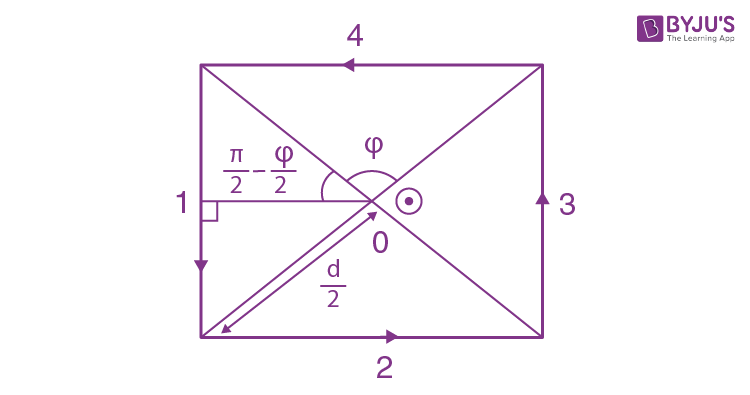Hence, the magnitude of total magnetic induction at O,

B0= B1 + B2+ B3 + B4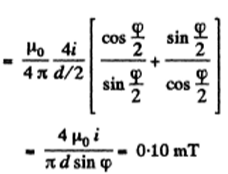4. A current l = 5.0 A flows along a thin wire shaped as shown in the figure. The radius of a curved part of the wire is equal to R = 120 mm, the angle 2φ = 90°. Find the magnetic induction of the field at point O.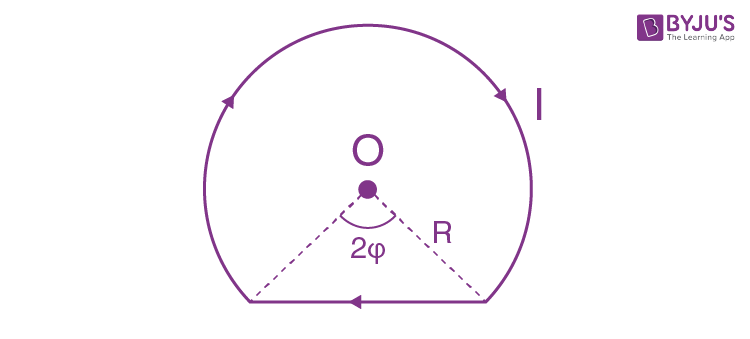Solution:

1. Magnetic induction due to the arc segment at O,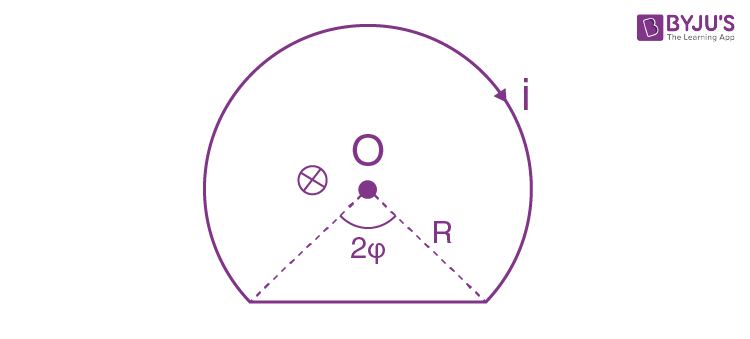${B}_{arc}=\frac{\mu _{0}}{4\pi }\frac{i}{R}(2\pi -2\phi )$

And magnetic induction due to the line segment at O

${B}_{line}=\frac{\mu _{0}}{4\pi }\frac{i}{R\cos \phi }(2\sin\phi )$

So, total magnetic induction at O

${B}_{0}={B}_{arc}+{B}_{line}=\frac{\mu _{0}}{2\pi }\frac{i}{R}(\pi -phi+\tan \phi)= 28\mu T$

5. Find the magnetic induction of the field at the point O of a loop with current I, whose shape is illustrated

1. (a) in Figure a, the radii a and b, as well as the angle φ are known;

2. (b) in Figure b, the radius a and the side b are known.

Solution:

1. From Biot -Savart law

$dB=\frac{\mu _{0}}{2\pi }i(\frac{d\vec{l}.\vec{r}}{r^{3}})$

So, magnetic field induction due to the segment 1 at O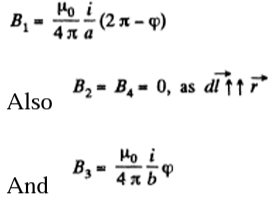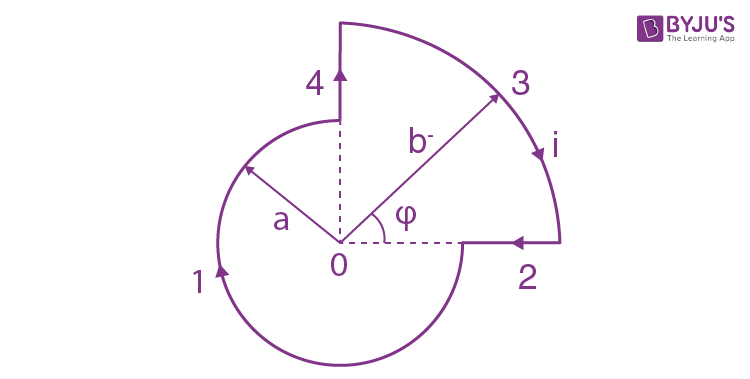B0= B1 + B2+ B3 + B4

$dB=\frac{\mu _{0}}{4\pi }i\left [ \frac{2\pi -\phi }{a} +\frac{\phi }{b}\right ]$

$B_{1}=\frac{\mu _{0}i}{4\pi a }\frac{3\pi }{a}$

B2 = 0

$B_{3}=B_{4}=\frac{\mu _{0}i}{4\pi b }sin 45^{0}$

And B5 = 0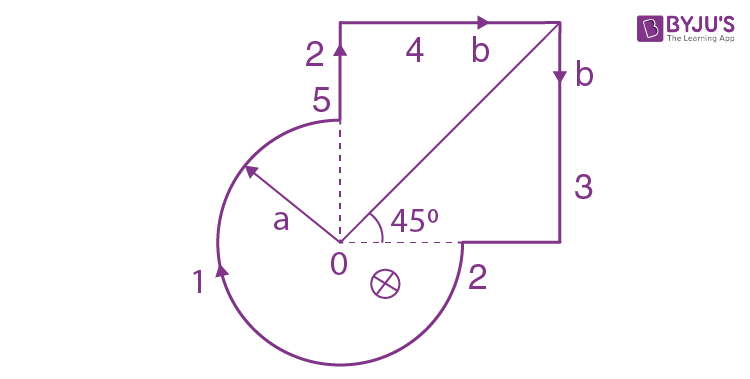And B5 = 0

B0= B1 + B2+ B3 + B4+B5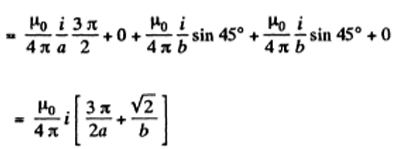6. A current I flows along a lengthy thin-walled tube of radius R with the longitudinal slit of width h. Find the induction of the magnetic field inside the tube under the condition h ≪ R.

Solution:

1. The thin-walled tube with a longitudinal slit can be considered equivalent to a full tube and a strip carrying the same current density in the opposite direction. Inside the tube, the former does not contribute so the total magnetic field is simply that due to the strip. It is

$B=\frac{\mu _{0}}{2\pi }\frac{(\frac{I}{2}\pi R)h}{r}=\frac{\mu _{o}Ih}{4\pi^{2}r}$

Where r is the distance of the field point from the strip.

7. A current I flows in a long straight wire with a cross-section having the form of a thin half-ring of radius R (Figure given). Find the induction of the magnetic field at point O.Solution:

1. First of all, let us find out the direction of the $\vec{B}$ at point O. For this purpose, we divide the entire conductor into elementary fragments with current di. It is obvious that the sum of any two symmetric fragments gives a resultant along $\vec{B}$ shown in the figure and consequently $\vec{B}$ will also be directed as shown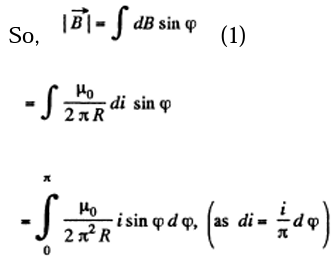Hence,

$B=\frac{\mu _{0}i}{\pi ^{2}R}$

8. Find the magnetic induction of the field at the point O if a current-carrying wire has the shape shown in Figure a, b, c. The radius of the curved part of the wire is R, the linear parts are assumed to be very long.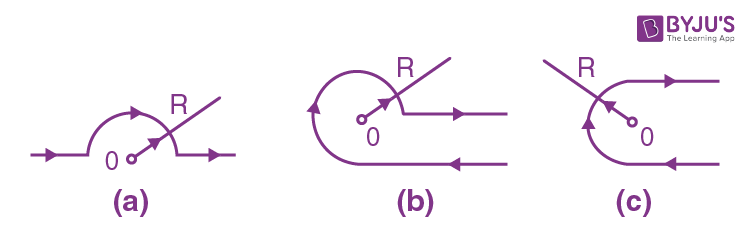Solution:

1. (a) From symmetry

B0= B1 + B2+ B3

$0+\frac{\mu _{0}}{4\pi}\frac{i}{R}\pi + 0=\frac{\mu _{0}}{4\pi}\frac{i}{R}$

(b) From symmetry

B0= B1 + B2+ B3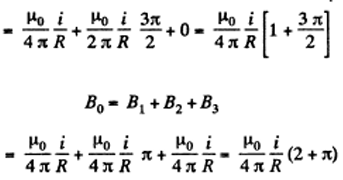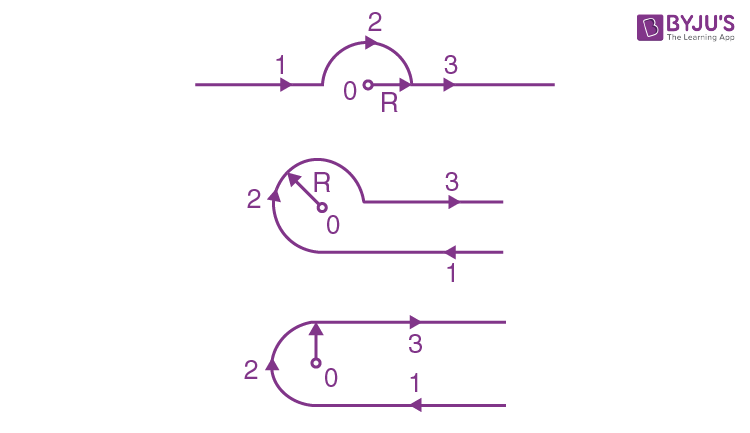9. A very long wire carrying a current I = 5.0 A is bent at right angles. Find the magnetic induction at a point lying on a perpendicular to the wire, drawn through the point of bending, at a distance l = 35 cm from it.

Solution:

1. $\vec{B}_{0}=\vec{B}_{1}+\vec{B}_{2}$

$\left | \vec{B}_{0} \right |=\frac{\mu _{0}i}{4\pi l}\sqrt{2}=2.0\mu T$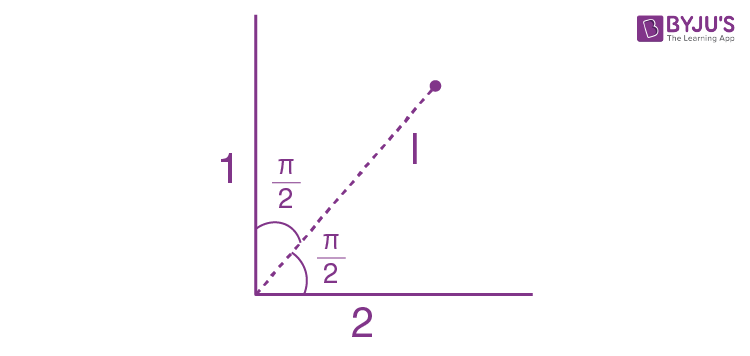10. Find the magnetic induction at point 0 if the wire carrying a current I = 8.0A has the shape shown in Figure a, b,c. The radius of the curved part of the wire is R = 100 mm, the linear parts of the wire are very long.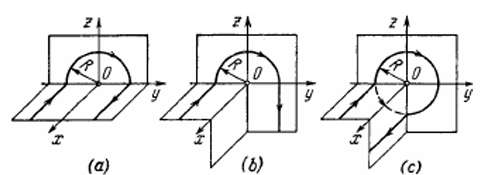Solution:

1. (a) $\vec{B}_{0}=\vec{B}_{1}+\vec{B}_{2}+\vec{B}_{3}$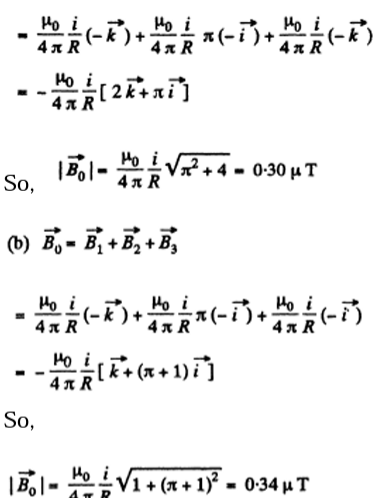(c) Here using the law of parallel resistances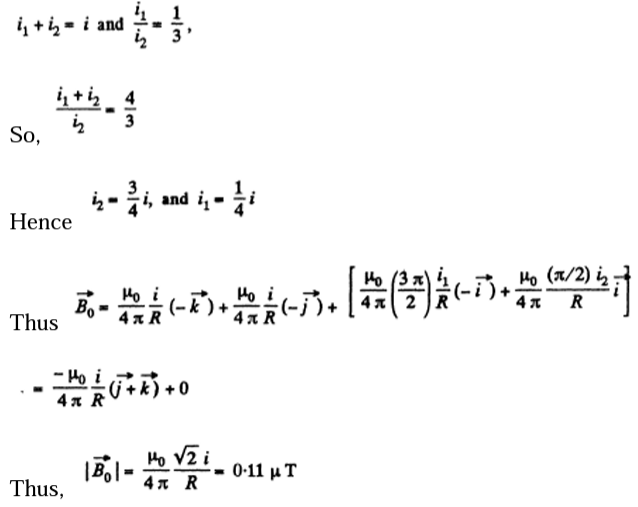11. Find the magnitude and direction of the magnetic induction vector B

1. (a) of an infinite plane carrying a current of linear density i; the vector i is the same at all points of the plane;

2. (b) of two parallel infinite planes carrying currents of linear densities i and- i. The vectors i and -i are constant at all points of the corresponding planes.

Solution:

1. (a) We apply the circulation theorem as shown. The current is vertically upwards in the plane and the magnetic field is horizontal and parallel to the file plane.

$\phi =\vec{B}.d\vec{l}=2Bl=\mu _{0}il$ or $B=\frac{\mu _{0}i}{2}$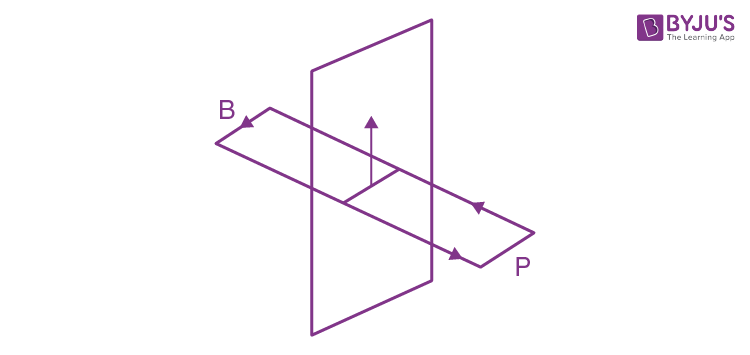(b) Each plane contributes $\frac{\mu _{0}i}{2}$ between the planes and outside the plane that cancel. Thus,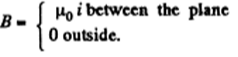12. A uniform current of density j flows inside an infinite plate of thickness 2d parallel to its surface. Find the magnetic induction induced by this current as a function of the distance x from the median plane of the plate. The magnetic permeability is assumed to be equal to unity both inside and outside the plate.

Solution:

1. We assume that the current flows perpendicular paper, by circulation theorem

$2Bdl=\mu _{0}(2x dl)j$

$2Bdl=\mu _{0}xj\left | x \right |\leq d$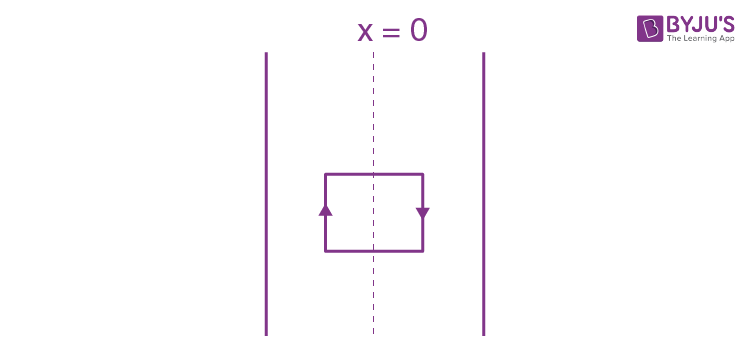Outside

$2Bdl=\mu _{0}(2d dl)j$

$2Bdl=\mu _{0}dj\left | x \right |\geq d$

13. A direct current I flows along a long straight wire. From the point O (Figure) the current spreads radially all perpendicular over an infinite conducting plane to the wire. Find the magnetic induction at all points of space.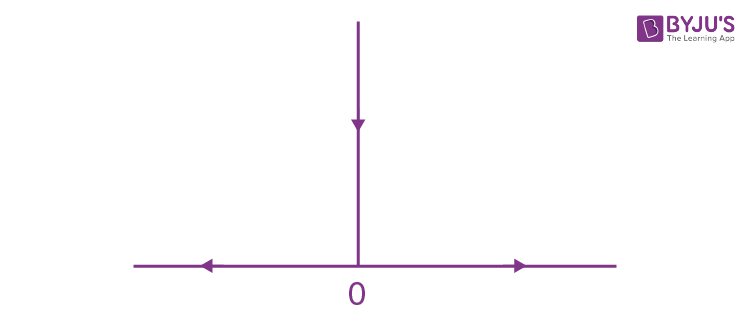Solution:

1. It is easy to convince oneself that both in the regions. 1 and 2, there can only be a circuital magnetic field (i.e. the component Bφ). Any radial field in region 1 or any Bz, away from the current plane will imply a violation of Gauss’ law magnetostatics, of Bφ, must obviously be symmetrical about the straight wire. Then in 1,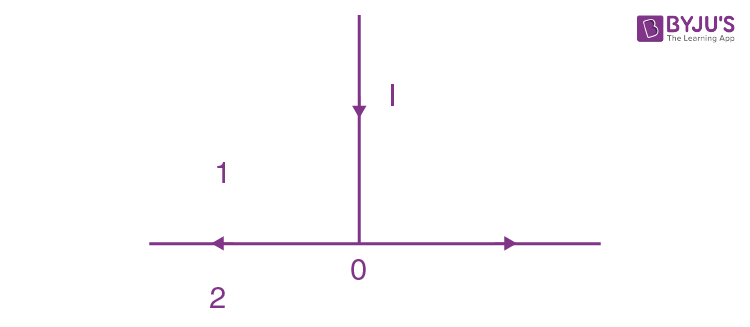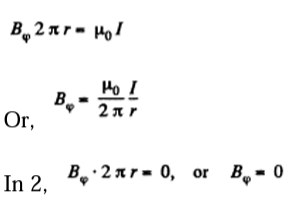14. A current I flows along a round loop. Find the integral $\int B dr$ along the axis of the loop within range from $-\infty \rightarrow +\infty$. Explain the result obtained.

Solution:

1. On the axis

$B=\frac{\mu _{0}IR^{2}}{2(R^{2}+x^{2})\frac{3}{2}}=B_{x}$ along the axis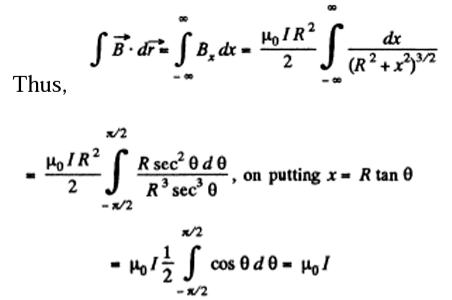The physical interpretation of this result is that $\int_{-\infty }^{+\infty}B_{x}$ dx can be thought of as the circulation of B over a closed-loop by imaging that the two ends of the axis are connected, by a line at infinity (e.g. a semicircle of infinite radius).

15. A direct current of density j flows along a round uniform straight wire with a cross-section radius R. Find the magnetic induction vector of this current at the point whose position relative to the axis of the wire is defined by a radius vector r. The magnetic permeability is assumed to be equal to unity throughout all the space.

Solution:

1. By circulation theorem inside the conductor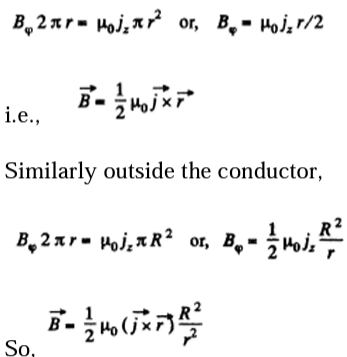16. Inside a long straight uniform wire of round crosssection there is a long round cylindrical cavity whose axis is parallel to the axis of the wire and displaced from the latter by a distance 1. A direct current of density j flows along the wire. Find the magnetic induction inside the cavity. Consider, in particular, the case I = 0.

Solution:

1. We can think of the given current which will be assumed uniform, as arising due to a negative current, flowing in the cavity, superimposed on the true current, everywhere including the cavity. Then from the previous problem, by superposition.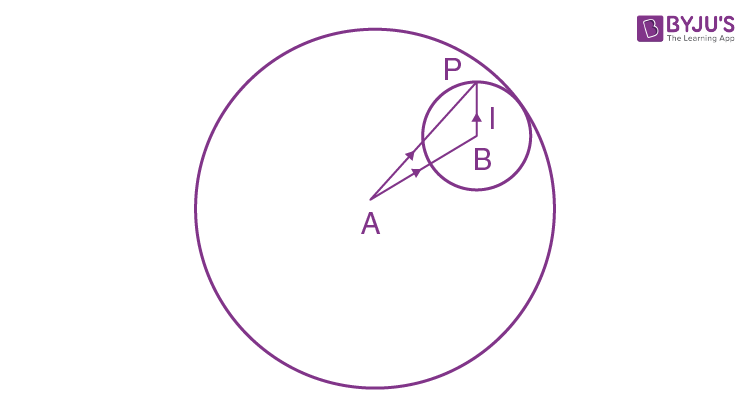$\vec{B}=\frac{1}{2}\mu _{0}\vec{j} (A\vec{p} - B\vec{p})\frac{1}{2}\mu _{0}\vec{j}\vec{l}$

If $\vec{l}$ vanishes so that the cavity is concentric with the conductor, there is no magnetic field in the cavity.

17. Find the current density as a function of distance r from the axis of a radially symmetrical parallel stream of electrons if the magnetic induction inside the stream varies as B = brα, where b and α are positive constants.

Solution:

1. By circulation theorm

$B_{\phi} .2\pi r=\mu _{0}\int_{0}^r{j(r')2\pi r'dr'}$

or using Bφ = brα inside the stream

$B_{r}^{\alpha +1} .2\pi r=\mu _{0}\int_{0}^r{j(r') r'dr'}$

So by differentiation,

${\alpha +1}B_{r}^\alpha=\mu _{0}{j(r) r}$

Hence,

$j(r) =\frac{b(\alpha +1)}{\mu _{0}}r^{\alpha -1}$

18. A single-layer coil (solenoid) has length l and cross-section radius R, A number of turns per unit length is equal to n. Find the magnetic induction at the centre of the coil when a current I flows through it.

Solution:

1. On the surface of the solenoid there is a surface current density

$\vec{j_{s}}=nIe\hat{\phi }$

Then

$\vec{B}=\frac{\mu _{o}}{4\pi }nI\int R d\phi dz\frac{\hat{e}_{\phi } \vec{r_{o}}}{r_{o}^{3}}$

When $\vec{r_{0}}$ is the vector from the current element to the field point, which is the centre of the solenoid.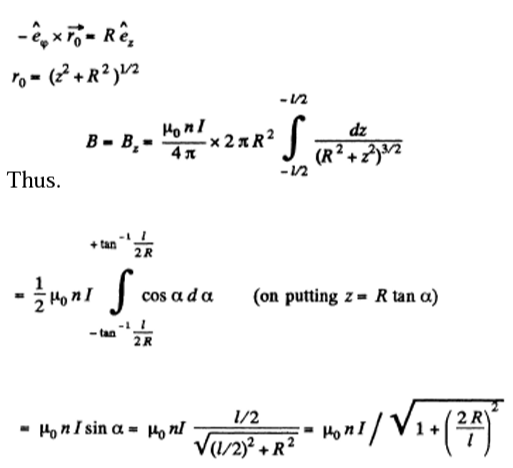Question 19. A very long straight solenoid has a crosssection radius R and n turns per unit length. A direct current I flows through the solenoid. Suppose that x is the distance from the end of the solenoid, measured along its axis. Find:

(a) the magnetic induction B on the axis as a function of x; draw an approximate plot of B vs the ratio x/R;

(b) the distance xo to the point on the axis at which the value of B differs by η = 1% from that in the middle section of the solenoid.

Solution:

1. We proceed exactly as in the previous problem. Then (a) the magnetic induction on the axis at a distance x from one end is clearly,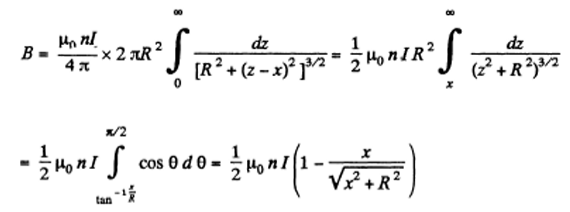x > 0 means that the field point is outside the solenoid. B then falls with x. x < 0 means that the field point gets more and more inside the solenoid. B then increases with (x) and eventually becomes constant, equal to μonI.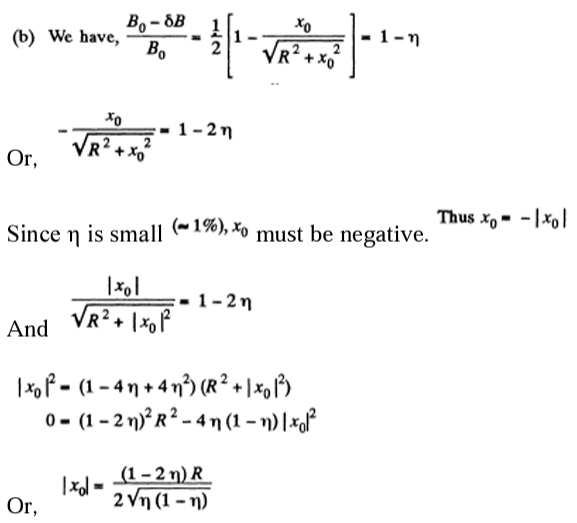20. A thin conducting strip of width h = 2.0 cm is tightly wound in the shape of a very long coil with a crosssection radius R = 2.5 cm to make a single-layer straight solenoid. A direct current I = 5.0 A flows through the strip. Find the magnetic induction inside and outside the solenoid as a function of the distance r from its axis.

Solution:

1. If the strip is tightly wound, it must have a pitch of A. This means that the current will flow obliquely, partly along $\hat{e}_{\phi }$ $\hat{e}_{\phi }$ $\hat{e}_{z }$. Obviously, the surface current density is,

$\vec{J}_{s}=\frac{I}{h}\left [ \sqrt{1-(\frac{h}{2\pi R^{2}})} \hat{e}_{\phi }+\frac{h}{2\pi R}\hat{e}_{z}\right ]$

$\vec{J}_{s}=\frac{I}{h}\left [ \sqrt{1-(\frac{h}{2\pi R^{2}})} \hat{e}_{\phi }+\frac{h}{2\pi R}\hat{e}_{z}\right ]$

By comparison with the case of a solenoid and a hollow straight conductor, we see that field inside the coil

$\mu _{0}\frac{I}{h}\sqrt{1-(\frac{h}{2\pi R)^{2}}}$

Outside, only the other term contributes, so